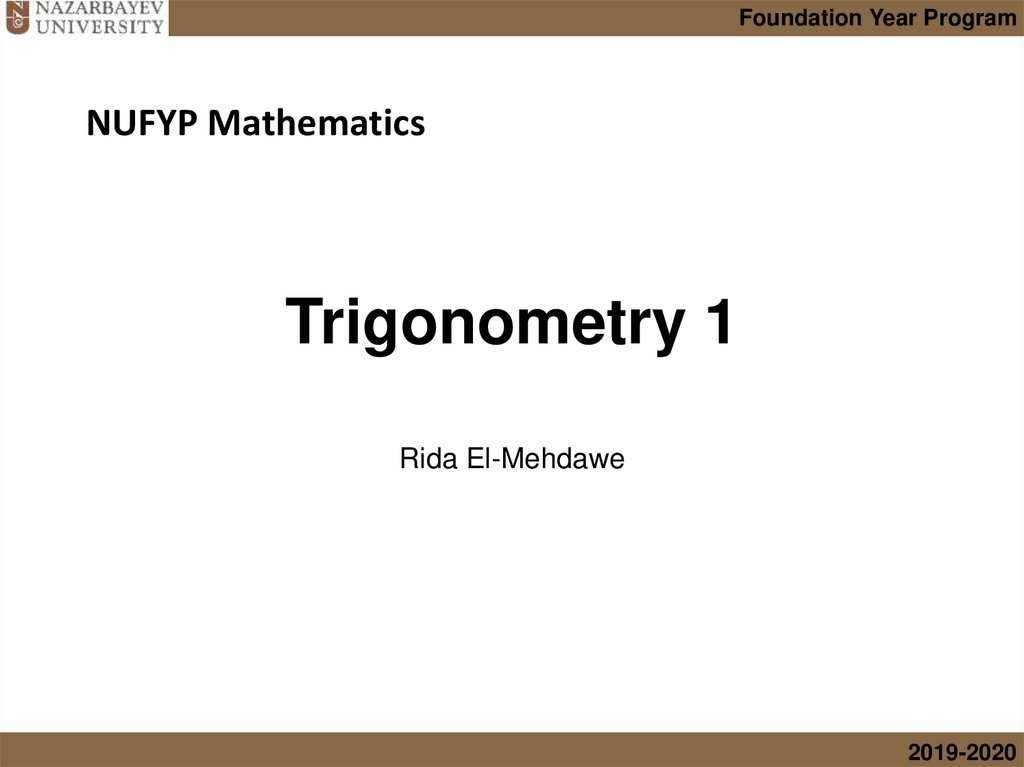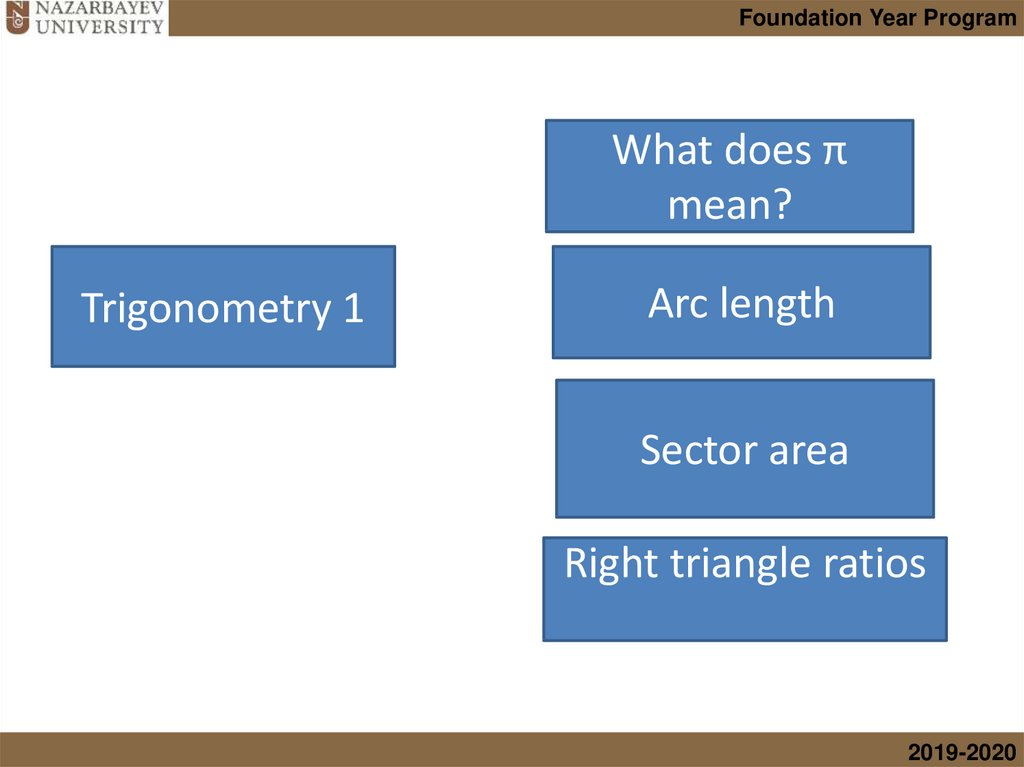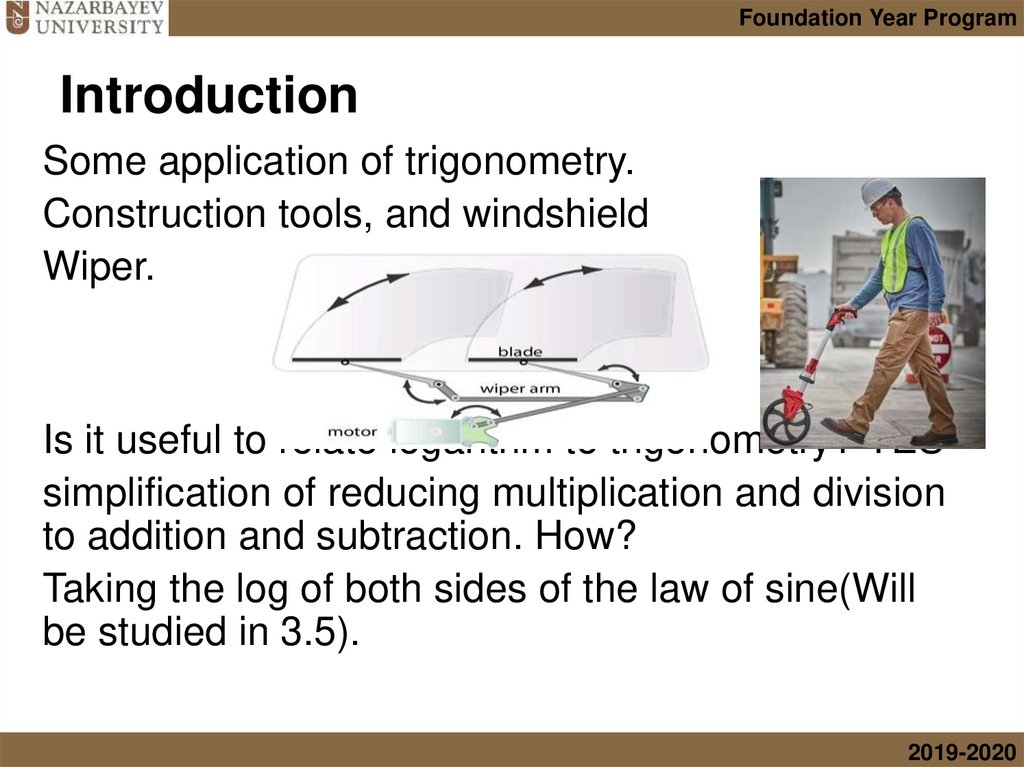# Trigonometry 1

## 1. Trigonometry 1

Foundation Year Program
NUFYP Mathematics
Trigonometry 1
Rida El-Mehdawe
2019-2020

## 2.

Foundation Year Program
What does π
mean?
Trigonometry 1
Arc length
Sector area
Right triangle ratios
2019-2020

## 3. Introduction

Foundation Year Program
Introduction
Some application of trigonometry.
Construction tools, and windshield
Wiper.
Is it useful to relate logarithm to trigonometry? YES
simplification of reducing multiplication and division
to addition and subtraction. How?
Taking the log of both sides of the law of sine(Will
be studied in 3.5).
2019-2020

## 4. Basic definitions

Foundation
Foundation Year
Year Program
Basic definitions
• If the arc AB has length